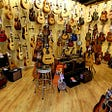# Color Separation in an Image using KMeans Clustering using Python

Color Separation in an image is a process of separating colors in the image. This process is done through the KMeans Clustering Algorithm.K-means clustering is one of the simplest and popular unsupervised machine learning algorithms.K-means algorithms identify k number of centroids, and then allocates every data point to the nearest cluster, while keeping the centroids as small as possible. The ‘means’ in the K-means refers to averaging of the data; that is, finding the centroid.

# Image Color Separation:-

We will be clustering the pixel intensities of an RGB image. Given an MXN size image, we thus have MxN pixels, each consisting of three components: Red, Green, and Blue respectively. We will treat these MxN pixels as our data points and cluster them using k-means. Pixels that belong to a given cluster will be more similar in color than pixels belonging to a separate cluster. One caveat of k-means is that we need to specify the number of clusters we want to generate ahead of time.

Here I am demonstrating the way by considering the following image.

1. Import Libraries:-

Firstly we need to import the basic libraries like NumPy, pandas, matplotlib, OpenCV, and Kmeans module from sklearn cluster library and some other libraries.

Now we need to load the image and convert the image into an image array using OpenCV.

The output of the above code is an image shown below:-

3. Converting from BGR to RGB:-

Generally when we are reading images through OpenCV then it reads in BGR format. So We need to convert it from BGR to RGB.

Below is the image showing both BGR and Converted RGB Image.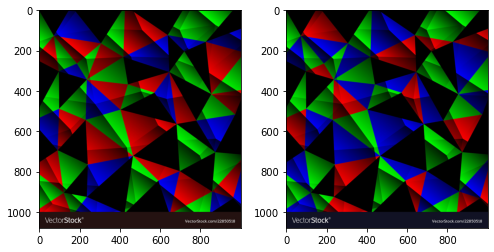Left is an image with BGR Format and Right is converted image with RGB Format.

4. Reshaping the image:-

We require an RGB pixel intensity to cluster. If the image contains MXN pixels then the shape of the image is (M, N,3) so we are reshaping image array to shape (M*N,3).

5. Implementing the KMeans Algorithm:-

Here we can fix no of clusters or can find using elbow point for simplicity firstly we are fixing no of clusters to 5 and fit the image.

6. Determining Labels:-

The training process is over. Now we need to determine the labels for each RGB pixel intensities.

The output is:-

`array([2, 2, 2, ..., 2, 2, 2], dtype=int32)`

7. Determining Centroids of Clusters:-

Now we need to determine the centroids of clusters for RGB pixel intensities.

The output of the above code is

`array([[  4.94156198, 194.05106194,   5.66103601],[  0.99513509,   1.17261867, 159.92115244],[  6.9601887 ,   4.80056827, 11.83784188],[162.89434822,   0.61579429,   0.99808952],[  0.9943044 ,  104.50070668,   1.50686636]])`

8. Calculating the Percentages:-

Now we need the calculate the percentage of each cluster that constitutes.

The output of the above code is:-

`[0.11026111111111112,  0.2055537037037037,  0.42694444444444446,  0.12552592592592593,  0.1317148148148148]`

9. Plotting a Pie chart:-

Now we got percentages of each color that constitutes in the image. The following colors are in the centroid list in the form of an RGBA format. Generally, the rgba values are from 0 to 1 so we need to divide each value in centroid to 255 and plot a pie chart using these percentages and colors.

The output of the above code is an image generated below:-

Now we got the colors in the image using KMeans Clustering here we fixed the k value. Generally for simplicity purposes, we constrain k but there is a process to find optimal k by finding elbow point.

## Elbow Method:-

The Elbow Method is one of the most popular methods to determine this optimal value of k.

We now define the following:-

Inertia: It is the sum of squared distances of samples to their closest cluster center.

We iterate the values of k from 1 to n and calculate the values of distortions for each value of k and calculate the distortion and inertia for each value of k in the given range.

The output of the above code is inertia value for each k from 1 to 20 values.

`[13107924711.978294,  8433820366.758524,  4507406394.104001,  1906016765.3226116,  1479036935.644275,  1167644004.3794537,  924556364.7463465,  677398333.8710563,  529621680.23856145,  424009662.682969,  359435146.0253957,  297634711.33955854,  252723281.89632946,  220941246.79365787,  193110661.4033114,  172814060.41467503,  155705229.97394535,  142992728.61409003,  131053276.08425024,  118995898.8872379]`

Plotting the inertia values with respect to each k as the graph.

The output is a plot shown below

Here from k=4 onwards in the above plot the there is no significant change in the value of inertia. So the elbow point is k=4.

Consider the same example with k=4 and repeat the process.

The output of the above code is an image shown below:-

Thus it improved the accuracy of the separation of colors. In this way, we can achieve the separation of colors in an image using KMeans Clustering.

The following are some examples generated by this method:-

Check Out my article on image augmentation using Keras here

https://medium.com/analytics-vidhya/understanding-image-augmentation-using-keras-tensorflow-a6341669d9ca

--

--

--

## More from Analytics Vidhya

Analytics Vidhya is a community of Analytics and Data Science professionals. We are building the next-gen data science ecosystem https://www.analyticsvidhya.com

## Computer Vision With OpenStreetMap and SpaceNet — A Comparison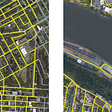## Solving Quora duplicate questions challenge the right way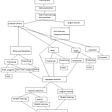## Predictive Maintenance with ResNet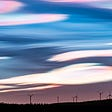## Spell Correction using CNN 1d and BILSTM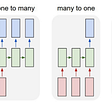## Fine Tuning your Deep Learning Model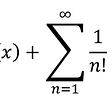## A beginner’s guide to NumPy with Sigmoid, ReLu and Softmax activation functions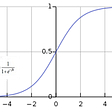## Few Shot Learning from Scratch## Introduction to Turing Learning and GANs## Sai Durga Kamesh Kota

Google DSC ML Lead | AI Practitioner🤖 | 3️⃣ rd year undergrad 🎓 in CSE 💻 at NIT Patna | Tech Blogger📝

## House Price Prediction using Machine Learning with Python## Understanding Figure object in Matplotlib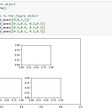## Random Forest Classifier Explained## Create your own dataset in pandas: Music shops chain example